Elementary Algebra

# 8.6Solve Rational Equations

Elementary Algebra8.6 Solve Rational Equations

### Learning Objectives

By the end of this section, you will be able to:

• Solve rational equations
• Solve a rational equation for a specific variable

### Be Prepared 8.6

Before you get started, take this readiness quiz.

If you miss a problem, go back to the section listed and review the material.

1. Solve: $16x+12=13.16x+12=13.$
If you missed this problem, review Example 2.48.
2. Solve: $n2−5n−36=0.n2−5n−36=0.$
If you missed this problem, review Example 7.73.
3. Solve for $yy$ in terms of $xx$: $5x+2y=105x+2y=10$ for $y.y.$
If you missed this problem, review Example 2.65.

After defining the terms expression and equation early in Foundations, we have used them throughout this book. We have simplified many kinds of expressions and solved many kinds of equations. We have simplified many rational expressions so far in this chapter. Now we will solve rational equations.

The definition of a rational equation is similar to the definition of equation we used in Foundations.

### Rational Equation

A rational equation is two rational expressions connected by an equal sign.

You must make sure to know the difference between rational expressions and rational equations. The equation contains an equal sign.

$Rational ExpressionRational Equation18x+1218x+12=14y+6y2−36y+6y2−36=y+11n−3+1n+41n−3+1n+4=15n2+n−12Rational ExpressionRational Equation18x+1218x+12=14y+6y2−36y+6y2−36=y+11n−3+1n+41n−3+1n+4=15n2+n−12$

### Solve Rational Equations

We have already solved linear equations that contained fractions. We found the LCD of all the fractions in the equation and then multiplied both sides of the equation by the LCD to “clear” the fractions.

Here is an example we did when we worked with linear equations: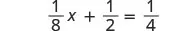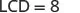We multiplied both sides by the LCD.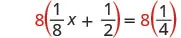Then we distributed.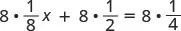We simplified—and then we had an equation with no fractions.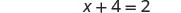Finally, we solved that equation.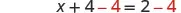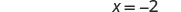We will use the same strategy to solve rational equations. We will multiply both sides of the equation by the LCD. Then we will have an equation that does not contain rational expressions and thus is much easier for us to solve.

But because the original equation may have a variable in a denominator we must be careful that we don’t end up with a solution that would make a denominator equal to zero.

So before we begin solving a rational equation, we examine it first to find the values that would make any denominators zero. That way, when we solve a rational equation we will know if there are any algebraic solutions we must discard.

An algebraic solution to a rational equation that would cause any of the rational expressions to be undefined is called an extraneous solution.

### Extraneous Solution to a Rational Equation

An extraneous solution to a rational equation is an algebraic solution that would cause any of the expressions in the original equation to be undefined.

We note any possible extraneous solutions, c, by writing $x≠cx≠c$ next to the equation.

### Example 8.59

#### How to Solve Equations with Rational Expressions

Solve: $1x+13=56.1x+13=56.$

### Try It 8.117

Solve: $1y+23=15.1y+23=15.$

### Try It 8.118

Solve: $23+15=1x.23+15=1x.$

The steps of this method are shown below.

### How To

#### Solve equations with rational expressions.

1. Step 1. Note any value of the variable that would make any denominator zero.
2. Step 2. Find the least common denominator of all denominators in the equation.
3. Step 3. Clear the fractions by multiplying both sides of the equation by the LCD.
4. Step 4. Solve the resulting equation.
5. Step 5.
Check.
• If any values found in Step 1 are algebraic solutions, discard them.
• Check any remaining solutions in the original equation.

We always start by noting the values that would cause any denominators to be zero.

### Example 8.60

Solve: $1−5y=−6y2.1−5y=−6y2.$

### Try It 8.119

Solve: $1−2a=15a2.1−2a=15a2.$

### Try It 8.120

Solve: $1−4b=12b2.1−4b=12b2.$

### Example 8.61

Solve: $53u−2=32u.53u−2=32u.$

### Try It 8.121

Solve: $1x−1=23x.1x−1=23x.$

### Try It 8.122

Solve: $35n+1=23n.35n+1=23n.$

When one of the denominators is a quadratic, remember to factor it first to find the LCD.

### Example 8.62

Solve: $2p+2+4p−2=p−1p2−4.2p+2+4p−2=p−1p2−4.$

### Try It 8.123

Solve: $2x+1+1x−1=1x2−1.2x+1+1x−1=1x2−1.$

### Try It 8.124

Solve: $5y+3+2y−3=5y2−9.5y+3+2y−3=5y2−9.$

### Example 8.63

Solve: $4q−4−3q−3=1.4q−4−3q−3=1.$

### Try It 8.125

Solve: $2x+5−1x−1=1.2x+5−1x−1=1.$

### Try It 8.126

Solve: $3x+8−2x−2=1.3x+8−2x−2=1.$

### Example 8.64

Solve: $m+11m2−5m+4=5m−4−3m−1.m+11m2−5m+4=5m−4−3m−1.$

### Try It 8.127

Solve: $x+13x2−7x+10=6x−5−4x−2.x+13x2−7x+10=6x−5−4x−2.$

### Try It 8.128

Solve: $y−14y2+3y−4=2y+4+7y−1.y−14y2+3y−4=2y+4+7y−1.$

The equation we solved in Example 8.64 had only one algebraic solution, but it was an extraneous solution. That left us with no solution to the equation. Some equations have no solution.

### Example 8.65

Solve: $n12+n+33n=1n.n12+n+33n=1n.$

### Try It 8.129

Solve: $x18+x+69x=23x.x18+x+69x=23x.$

### Try It 8.130

Solve: $y+55y+y15=1y.y+55y+y15=1y.$

### Example 8.66

Solve: $yy+6=72y2−36+4.yy+6=72y2−36+4.$

### Try It 8.131

Solve: $xx+4=32x2−16+5.xx+4=32x2−16+5.$

### Try It 8.132

Solve: $yy+8=128y2−64+9.yy+8=128y2−64+9.$

### Example 8.67

Solve: $x2x−2−23x+3=5x2−2x+912x2−12.x2x−2−23x+3=5x2−2x+912x2−12.$

### Try It 8.133

Solve: $y5y−10−53y+6=2y2−19y+5415y2−60.y5y−10−53y+6=2y2−19y+5415y2−60.$

### Try It 8.134

Solve: $z2z+8−34z−8=3z2−16z−68z2+8z−64.z2z+8−34z−8=3z2−16z−68z2+8z−64.$

### Solve a Rational Equation for a Specific Variable

When we solved linear equations, we learned how to solve a formula for a specific variable. Many formulas used in business, science, economics, and other fields use rational equations to model the relation between two or more variables. We will now see how to solve a rational equation for a specific variable.

We’ll start with a formula relating distance, rate, and time. We have used it many times before, but not usually in this form.

### Example 8.68

Solve: $DT=RforT.DT=RforT.$

### Try It 8.135

Solve: $AL=WAL=W$ for $L.L.$

### Try It 8.136

Solve: $FA=MFA=M$ for $A.A.$

Example 8.69 uses the formula for slope that we used to get the point-slope form of an equation of a line.

### Example 8.69

Solve: $m=x−2y−3fory.m=x−2y−3fory.$

### Try It 8.137

Solve: $y−2x+1=23y−2x+1=23$ for $x.x.$

### Try It 8.138

Solve: $x=y1−yx=y1−y$ for $y.y.$

Be sure to follow all the steps in Example 8.70. It may look like a very simple formula, but we cannot solve it instantly for either denominator.

### Example 8.70

Solve $1c+1m=1forc.1c+1m=1forc.$

### Try It 8.139

Solve: $1a+1b=c1a+1b=c$ for $a.a.$

### Try It 8.140

Solve: $2x+13=1y2x+13=1y$ for $y.y.$

### Section 8.6 Exercises

#### Practice Makes Perfect

Solve Rational Equations

In the following exercises, solve.

303.

$1 a + 2 5 = 1 2 1 a + 2 5 = 1 2$

304.

$5 6 + 3 b = 1 3 5 6 + 3 b = 1 3$

305.

$5 2 − 1 c = 3 4 5 2 − 1 c = 3 4$

306.

$6 3 − 2 d = 4 9 6 3 − 2 d = 4 9$

307.

$4 5 + 1 4 = 2 v 4 5 + 1 4 = 2 v$

308.

$3 7 + 2 3 = 1 w 3 7 + 2 3 = 1 w$

309.

$7 9 + 1 x = 2 3 7 9 + 1 x = 2 3$

310.

$3 8 + 2 y = 1 4 3 8 + 2 y = 1 4$

311.

$1 − 2 m = 8 m 2 1 − 2 m = 8 m 2$

312.

$1 + 4 n = 21 n 2 1 + 4 n = 21 n 2$

313.

$1 + 9 p = −20 p 2 1 + 9 p = −20 p 2$

314.

$1 − 7 q = −6 q 2 1 − 7 q = −6 q 2$

315.

$1 r + 3 = 4 2 r 1 r + 3 = 4 2 r$

316.

$3 t − 6 = 1 t 3 t − 6 = 1 t$

317.

$5 3 v − 2 = 7 4 v 5 3 v − 2 = 7 4 v$

318.

$8 2 w + 1 = 3 w 8 2 w + 1 = 3 w$

319.

$3 x + 4 + 7 x − 4 = 8 x 2 − 16 3 x + 4 + 7 x − 4 = 8 x 2 − 16$

320.

$5 y − 9 + 1 y + 9 = 18 y 2 − 81 5 y − 9 + 1 y + 9 = 18 y 2 − 81$

321.

$8 z − 10 + 7 z + 10 = 5 z 2 − 100 8 z − 10 + 7 z + 10 = 5 z 2 − 100$

322.

$9 a + 11 + 6 a − 11 = 7 a 2 − 121 9 a + 11 + 6 a − 11 = 7 a 2 − 121$

323.

$1 q + 4 − 7 q − 2 = 1 1 q + 4 − 7 q − 2 = 1$

324.

$3 r + 10 − 4 r − 4 = 1 3 r + 10 − 4 r − 4 = 1$

325.

$1 t + 7 − 5 t − 5 = 1 1 t + 7 − 5 t − 5 = 1$

326.

$2 s + 7 − 3 s − 3 = 1 2 s + 7 − 3 s − 3 = 1$

327.

$v − 10 v 2 − 5 v + 4 = 3 v − 1 − 6 v − 4 v − 10 v 2 − 5 v + 4 = 3 v − 1 − 6 v − 4$

328.

$w + 8 w 2 − 11 w + 28 = 5 w − 7 + 2 w − 4 w + 8 w 2 − 11 w + 28 = 5 w − 7 + 2 w − 4$

329.

$x − 10 x 2 + 8 x + 12 = 3 x + 2 + 4 x + 6 x − 10 x 2 + 8 x + 12 = 3 x + 2 + 4 x + 6$

330.

$y − 3 y 2 − 4 y − 5 = 1 y + 1 + 8 y − 5 y − 3 y 2 − 4 y − 5 = 1 y + 1 + 8 y − 5$

331.

$z 16 + z + 2 4 z = 1 2 z z 16 + z + 2 4 z = 1 2 z$

332.

$a 9 + a + 3 3 a = 1 a a 9 + a + 3 3 a = 1 a$

333.

$b + 3 3 b + b 24 = 1 b b + 3 3 b + b 24 = 1 b$

334.

$c + 3 12 c + c 36 = 1 4 c c + 3 12 c + c 36 = 1 4 c$

335.

$d d + 3 = 18 d 2 − 9 + 4 d d + 3 = 18 d 2 − 9 + 4$

336.

$m m + 5 = 50 m 2 − 25 + 6 m m + 5 = 50 m 2 − 25 + 6$

337.

$n n + 2 = 8 n 2 − 4 + 3 n n + 2 = 8 n 2 − 4 + 3$

338.

$p p + 7 = 98 p 2 − 49 + 8 p p + 7 = 98 p 2 − 49 + 8$

339.

$q3q−9−34q+12q3q−9−34q+12$
$=7q2+6q+6324q2−216=7q2+6q+6324q2−216$

340.

$r3r−15−14r+20r3r−15−14r+20$
$=3r2+17r+4012r2−300=3r2+17r+4012r2−300$

341.

$s2s+6−25s+5s2s+6−25s+5$
$=5s2−3s−710s2+40s+30=5s2−3s−710s2+40s+30$

342.

$t6t−12−52t+10t6t−12−52t+10$
$=t2−23t+7012t2+36t−120=t2−23t+7012t2+36t−120$

Solve a Rational Equation for a Specific Variable

In the following exercises, solve.

343.

$C r = 2 π for r C r = 2 π for r$

344.

$I r = P for r I r = P for r$

345.

$V h = l w for h V h = l w for h$

346.

$2 A b = h for b 2 A b = h for b$

347.

$v + 3 w − 1 = 1 2 for w v + 3 w − 1 = 1 2 for w$

348.

$x + 5 2 − y = 4 3 for y x + 5 2 − y = 4 3 for y$

349.

$a = b + 3 c − 2 for c a = b + 3 c − 2 for c$

350.

$m = n 2 − n for n m = n 2 − n for n$

351.

$1 p + 2 q = 4 for p 1 p + 2 q = 4 for p$

352.

$3 s + 1 t = 2 for s 3 s + 1 t = 2 for s$

353.

$2 v + 1 5 = 1 2 for v 2 v + 1 5 = 1 2 for v$

354.

$6 x + 2 3 = 1 y for y 6 x + 2 3 = 1 y for y$

355.

$m + 3 n − 2 = 4 5 for n m + 3 n − 2 = 4 5 for n$

356.

$E c = m 2 for c E c = m 2 for c$

357.

$3 x − 5 y = 1 4 for y 3 x − 5 y = 1 4 for y$

358.

$R T = W for T R T = W for T$

359.

$r = s 3 − t for t r = s 3 − t for t$

360.

$c = 2 a + b 5 for a c = 2 a + b 5 for a$

#### Everyday Math

361.

House Painting Alain can paint a house in 4 days. Spiro would take 7 days to paint the same house. Solve the equation $14+17=1t14+17=1t$ for t to find the number of days it would take them to paint the house if they worked together.

362.

Boating Ari can drive his boat 18 miles with the current in the same amount of time it takes to drive 10 miles against the current. If the speed of the boat is 7 knots, solve the equation $187+c=107−c187+c=107−c$ for c to find the speed of the current.

#### Writing Exercises

363.

Why is there no solution to the equation $3x−2=5x−23x−2=5x−2$?

364.

Pete thinks the equation $yy+6=72y2−36+4yy+6=72y2−36+4$ has two solutions, $y=−6andy=4y=−6andy=4$. Explain why Pete is wrong.

#### Self Check

After completing the exercises, use this checklist to evaluate your mastery of the objectives of this section.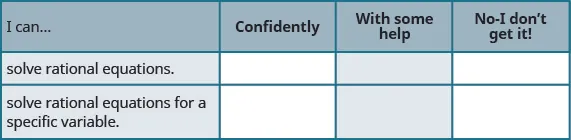After reviewing this checklist, what will you do to become confident for all objectives?

Order a print copy

As an Amazon Associate we earn from qualifying purchases.